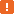# aqDateTime.Today Method### Description

Use the `aqDateTime.Today` method to get the current date. The resulting value holds only the date portion. To obtain both the current date and time, use the `Now` method. To obtain only the current time, use the `Time` method.

### Declaration

aqDateTime.Today()

 Result Date/Time

### Applies To

The method is applied to the following object:

### Result Value

A Date/Time value that holds the current date.In JavaScript, JScript, Python or DelphiScript, if you try to output the date and time returned by the `Today` method as a string, conversion takes place and the date and time are output in the native format for the selected language (`day/month/date/time/year` in JavaScript, JScript and DelphiScript, `year/month/day/time` in Python). For example, this occurs if you use the following code to post the current date to the test log:

JavaScript, JScript

function Test()
{
Log.Message("Today is " + aqDateTime.Today());
}

Python

``````def Test():
Log.Message("Today is " + str(aqDateTime.Today()))``````

VBScript

Sub Test
Log.Message("Today is" & aqDateTime.Today())
end sub

DelphiScript

function Test;
begin
Log.Message('Today is' + aqDateTime.Today);
end;

C++Script, C#Script

function Test()
{
Log["Message"]("Today is " + aqDateTime["Today"]());
}

To avoid such a conversion, you need to explicity specify the format in which time should be displayed. For example, you can use the following code:

JavaScript, JScript

function Test()
{
Log.Message("Today is " + aqConvert.DateTimeToFormatStr(aqDateTime.Today(), "%m/%d/%y"));
}

Python

``````def Test2():
Log.Message("Today is " + aqConvert.DateTimeToFormatStr(aqDateTime.Today(), "%m/%d/%y"))``````

VBScript

Sub Test
Log.Message("Today is" & aqConvert.DateTimeToFormatStr(aqDateTime.Today(), "%m/%d/%y"))
end sub

DelphiScript

function Test;
begin
Log.Message('Today is' + aqConvert.DateTimeToFormatStr(aqDateTime.Today(), '%m/%d/%y'));
end;

C++Script, C#Script

function Test()
{
Log["Message"]("Today is " + aqConvert["DateTimeToFormatStr"](aqDateTime["Today"](), "%m/%d/%y"));
}

### Example

The code below demonstrates how you can compare different date and time values.

JavaScript, JScript

function ComparingDates()
{
var currentDateAndTime = aqDateTime.Now();
var currentTime = aqDateTime.Time();
// The default date for currentTime is set to 12/30/1899
var currentDate = aqDateTime.Today();
// The default time for currentDate is set to 00:00:00

Log.Message(currentDateAndTime);
Log.Message(currentTime);
Log.Message(currentDate);

Log.Message(aqDateTime.Compare(currentDateAndTime, currentTime));
// 1, because currentDateAndTime is later then currentTime

Log.Message(aqDateTime.Compare(currentDateAndTime, currentDate));
// 1, because currentDateAndTime is later then currentDate

Log.Message(aqDateTime.Compare(currentTime, currentDate));
// -1, because currentTime is earlier then currentDate
}

Python

``````def ComparingDates():
currentDateAndTime = aqDateTime.Now()
currentTime = aqDateTime.Time()
# The default date for currentTime is set to 12/30/1899
currentDate = aqDateTime.Today()
# The default time for currentDate is set to 00:00:00
Log.Message(currentDateAndTime)
Log.Message(currentTime)
Log.Message(currentDate)
Log.Message(aqDateTime.Compare(currentDateAndTime, currentTime))
# 1, because currentDateAndTime is later then currentTime
Log.Message(aqDateTime.Compare(currentDateAndTime, currentDate));
# 1, because currentDateAndTime is later then currentDate
Log.Message(aqDateTime.Compare(currentTime, currentDate));
# -1, because currentTime is earlier then currentDate``````

VBScript

Sub ComparingDates
Dim currentDateAndTime, currentTime, currentDate

currentDateAndTime = aqDateTime.Now
currentTime = aqDateTime.Time
' The default date for currentTime is set to 12/30/1899
currentDate = aqDateTime.Today
' The default time for currentDate is set to 00:00:00

Log.Message(currentDateAndTime)
Log.Message(currentTime)
Log.Message(currentDate)

Log.Message(aqDateTime.Compare(currentDateAndTime, currentTime))
' 1, because currentDateAndTime is later then currentTime

Log.Message(aqDateTime.Compare(currentDateAndTime, currentDate))
' 1, because currentDateAndTime is later then currentDate

Log.Message(aqDateTime.Compare(currentTime, currentDate))
' -1, because currentTime is earlier then currentDate
End Sub

DelphiScript

procedure ComparingDates;
var currentDateAndTime, currentTime, currentDate;
begin
currentDateAndTime := aqDateTime.Now;
currentTime := aqDateTime.Time;
// The default date for currentTime is set to 12/30/1899
currentDate := aqDateTime.Today;
// The default time for currentDate is set to 00:00:00

Log.Message(currentDateAndTime);
Log.Message(currentTime);
Log.Message(currentDate);

Log.Message(aqDateTime.Compare(currentDateAndTime, currentTime));
// 1, because currentDateAndTime is later then currentTime

Log.Message(aqDateTime.Compare(currentDateAndTime, currentDate));
// 1, because currentDateAndTime is later then currentDate

Log.Message(aqDateTime.Compare(currentTime, currentDate));
// -1, because currentTime is earlier then currentDate
end;

C++Script, C#Script

function ComparingDates()
{
var currentDateAndTime = aqDateTime["Now"]();
var currentTime = aqDateTime["Time"]();
// The default date for currentTime is set to 12/30/1899
var currentDate = aqDateTime["Today"]();
// The default time for currentDate is set to 00:00:00

Log["Message"](currentDateAndTime);
Log["Message"](currentTime);
Log["Message"](currentDate);

Log["Message"](aqDateTime["Compare"](currentDateAndTime, currentTime));
// 1, because currentDateAndTime is later then currentTime

Log["Message"](aqDateTime["Compare"](currentDateAndTime, currentDate));
// 1, because currentDateAndTime is later then currentDate

Log["Message"](aqDateTime["Compare"](currentTime, currentDate));
// -1, because currentTime is earlier then currentDate
}